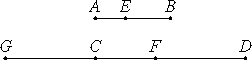# Proposition 7

If a number is that part of a number which a subtracted number is of a subtracted number, then the remainder is also the same part of the remainder that the whole is of the whole.

Let the number AB be that part of the number CD which AE subtracted is of CF subtracted.

I say that the remainder EB is also the same part of the remainder FD that the whole AB is of the whole CD.Let EB be the same part of CG that AE is of CF.

VII.5

Now since EB is the same part of CG that AE is of CF, therefore AB is the same part of GF that AE is of CF.

But, by hypothesis, AB is the same part of CD that AE is of CF, therefore AB is the same part of CD that it is of GF. Therefore GF equals CD.

Subtract CF from each. Then the remainder GC equals the remainder FD.

Now since EB is the same part of GC that AE is of CF, and GC equals FD, therefore EB is the same part of FD that AE is of CF.

But AB is the same part of CD that AE is of CF, therefore the remainder EB is the same part of the remainder FD that the whole AB is of the whole CD.

Therefore, if a number is that part of a number which a subtracted number is of a subtracted number, then the remainder is also the same part of the remainder that the whole is of the whole.

Q.E.D.

## Guide

This proposition is like VII.5 except it deals with subtraction instead of addition. It says division distributes over subtraction. Algebraically,

b/ne/n, = (be)/n.

This proposition is used in the next proposition and in VII.11.